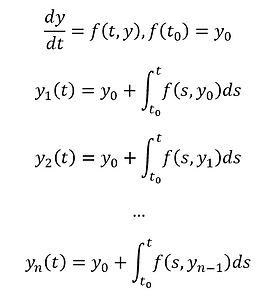Picard Iteration

Picard iteration is a technique to find a functional solution to an ODE through the generation of a power series.  There are many potential pitfalls to this technique that have revealed themselves as analysis has evolved and it turns out that numerically solving integrals is potentially more robust.  Still, in many circumstances, we can find a solution!

Now, suppose we have the following differential equation:                          .  Then, via an easy application of the fundamental theorem of calculus, we immediately have a solution of the form:                             .  But, of course, we have seen that not all our differential equations can be expressed entirely as functions of one variable.  As a result, it becomes necessary to be a bit more clever.  Note, that the general form of our ODE tends to be a function of both t and y and as a result, generally nonseparable.  Perhaps, though, we can use this for motivation?If we start with a function of t and y given by ƒ(t, y), then we can iteratively obtain the following.  Émile Picard, Augustin Cauchy, Ernst Lindelöf, and Rudolph Lipschitz all arrived at versions of or improvements on this theorem and its proof.  Many historical sources credit Cauchy with the initial version, but like many of his proofs, it was filled with tacit assumptions that most mathematicians today would find unacceptable.

It is also unlikely that he considered or understood the complete set of circumstances for this theorem to be applicable.  Note that the result of the iterative process is a power series.  This comes with all the potential pitfalls of a power series too!  Additionally, we need to make secondary considerations from functional analysis because our result is a function.  At this point, we should be familiar enough with ODEs to realize that we can arrive at the same functional solution with two different sets of initial conditions.  Is this always true in the case of Picard iteration?

Try solving the following example!First, we'll notice that the above differential equation is everywhere analytic and separable so it admits the solution                          and that that solution is precisely expressed everywhere by the power series we generated using Picard iteration.  Moreover, analyticity guarantees it is everywhere equal to this power series!

Let's consider a second easy example as well.  We'll explicitly compute just the first 3 iterates and note some of the potential issues that can occur here.